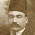## Thursday, January 26, 2012

### They say a watched boil never pots... or something

At standard atmospheric pressure, water boils at 212°F (100°C). But at lower atmospheric pressures, water boils at lower temperatures. Here's a short clip of some boiling water:

Some of you might remember the formula for converting temperature: [Temp(F)] = (9/5) * [Temp(C)] + 32
This isn't all that easy to remember - there are some odd fractions in there and to be honest - it's time consuming to multiply things by weird fractions. Here's a quick way to convert (roughly) from Fahrenheit to Celsius that I use:

A few reference figures:
•Water Boils at 212F, 100C
•"Standard" body temp is 98.6F, 37C
•Water Freezes at 32F, 0C
•"REALLY COLD" is -40F, -40C

Let's say you're on vacation and the weather report says it's a cool 10C outside. Well, you can estimate that 100F is about equivalent to 40C (close enough, plus we're simplifying the math) and 30F is about 0C. Now you have the range of temps in C and F. Think of it as the total length of the ruler - if we know how long each ruler is and know that one measurement is some percent of the total, that same percent of the other ruler is our target. The length of our ruler (or change in temp) from our estimate is 70 for fahrenheit, and the total change in C is 40. 10C is about 25% of that the "length" of our celsius ruler (10/40, 1/4th or 25%). So 25% (1/4th) of 70 is (half of a half, so half of 70 is 35, half of 35 is 17.5). Since our celsius temp is above freezing, we add that 17.5 to 32F and get 49.5 - rounded up to 50. Checking the calculator, that ends up being spot-on (10C = 50F).

We could use the boiling and freezing points of water - since not everyone can remember 37C as the body temp. Water's liquid range is 100 degrees for celsius, and 180 for fahrenheit. 10C is 10% of liquid water's celsius range (10/100, 1/10th, or 10%), so 10% of the fahrenheit range is 18. SInce it's above freezing, 18+32 = 50 degrees fahrenheit. Not bad, eh?

Oh, and be very, very careful when the TV weather personality says it's absolute zero outside. That's cold - this refers to an object with zero heat energy (simplistically speaking). That's -273.16°C. BRRR! What about fahrenheit? Okay, so we know 0C and 32F are equivalent and (interestingly) -40 is the same in F and C. So the range here is 40 degrees celsius and 72 degrees fahrenheit. Well, -273 is a little less than 7 times our range of 40. So for our range in fahrenheit, 7 x 70 (again, rounding down to nearest 10 to simplify the math in our heads) is 490. Since we're below freezing, we subtract 490 from 32 to get -458°F.

Wouldn't you know it, -273.15°C is -459.67°F. My mental conversion must be a little low because I rounded down in order to keep the math quick and easy. At those temperatures, are you going to worry about being less than two degrees off? Well, sure, if you're trying to form a Bose-Einstein condensate - but then you're not going to be rounding numbers to the nearest degree - or even worrying about english imperial units at all.

But in everyday life, you don't need a calculator all the time. Estimation is a powerful tool. If you need to be precise, use a machine. If you just need to get an idea, use your head. You always have that with you and it doesn't need batteries.

1.2.